# Continuous function facts for kids

Kids Encyclopedia Facts

In mathematics a function is said to be continuous if, roughly said, a small change in the input only causes a small change in the output. If this is not the case, the function is said to be discontinuous. Functions defined on the real numbers, with one input and one output variable, will show as an uninterrupted line (or curve). They can be drawn without lifting the pen. The definition given above was made by Augustin-Louis Cauchy.

Karl Weierstraß gave another definition of continuity: Suppose that there is a function f, defined on the real numbers. At the point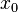$x_0$ the function will have the value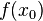$f(x_0)$. If the function is continuous at$x_0$, then for every value of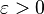$\varepsilon>0$ no matter how small it is, there is a value of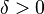$\delta > 0$, so that whenever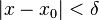$|x - x_0| < \delta$, that makes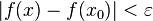$|f(x) - f(x_0)| < \varepsilon$. Intuitively: Given a point close to$x_0$ (called x), the absolute value of the difference between the two values of the function can be made arbitrarily small, if the point x is close enough to$x_0$.

There are also special forms of continuous, such as Lipschitz-continuous. A function is Lipschitz-continuous if there is a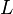$L$ with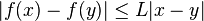$|f(x) - f(y)| \le L|x - y|$ for all x,y ∈ (a,b).

## Images for kidsContinuous function Facts for Kids. Kiddle Encyclopedia.